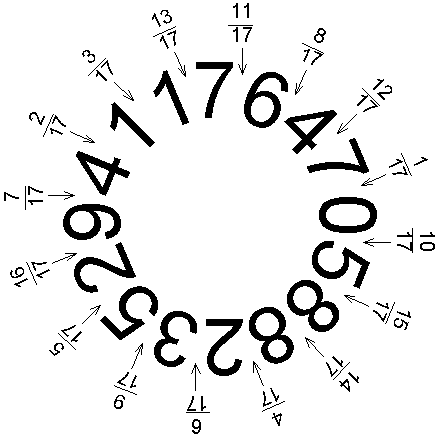This header plots the critical line of the Riemann Zeta Function.  A complete understanding wins a \$1,000,000 prize.
 . . .Main Links Orders Post Next Page Next + 10

I liked Long Division in school.  Is it still taught?  There's a neat little trick you can do involving repeating decimals.  Ask a friend for a number between 1 and 20.  Suppose they pick 5.  Have them calculate 5/23.  They will start the calculation on the left.
 0.21739130434  23)5.0000000000     4 6      40       23      170       161        90         69        210         207          30           23           70            69            100             92              80             69              110             92 "STOP!" you say suddenly.  If your friend is really into the division by now, they will probably be quite startled.  You continue, writing the numbers down as you speak. -- "I've done the whole thing in my head.  The next eleven digits are 78260869565, and then it starts repeating.  Check me, if you'd like." Indeed, 5/23 = .2173913043478260869565 2173913043478260869565 ... This fraction has a repeating decimal, or repetend. If your friend thinks you've memorized the numbers, you can repeat your trick with 7, 17, 19, 23, 29, 47, 59, 61, 97, 109, or 113.  What's the trick?  It's all based on a theorem proved in 1836 by E. Midy. 1/97 = 0. 010309278350515463917525773195876288659793814432 989690721649484536082474226804123711340206185567 and it repeats.  Add up each column to see the trick. For a prime p, if the repetend of 1/p has 2n digits, then digit (n+k) = 9 - digit (k).  So, once your friend has done the hard half of the work, you finish by just subtracting each digit from 9. For the above numbers, the length of the repetend is p-1.  Thus, you would stop your friend after (p-1)/2 digits.  These are known as long primes, or primes with 10 as a primitive root.  Whether there is an infinite number of these is an unsolved problem.
The repetend of the reciprocal of a long prime is multifunctional.  If you know 3/17, then finding 4/17 is easy.  The repetend is cyclic.If you prefer using an 8-digit calculator to long division, you can still calculate complete decimals by taking advantage of the cyclic property and the Midy property.  Suppose you needed 1/17.  Your calculator might only give you 0.0588235, and the last digit is questionable.  Take that one off, and write down the rest.  Below that, write the sequence given by the Midy method -- 941176.  Now, just multiply by a number of your choice.  It will give you more information to fill in the gaps.  Using this method, you should have no problem figuring out the repetend of 1/29.

Ignacio Ruiz de Conejo, Taus Brock-Nannestad, David Fowler, John Bollinger, Dick Saunders Jr, and Moorthy all sent answers.  Moorthy also pointed me to http://www.cs.rpi.edu/~moorthy/vm/ , which explains these more.